Breaking News
Home / Civil Engineering / How To Estimate The Roofing Sheet Requirement For a Building

# How To Estimate The Roofing Sheet Requirement For a Building

### How To Estimate The Roofing Sheet Requirement For a Building

Let us Calculate the Number Of Roofing Sheets required for the below-given building.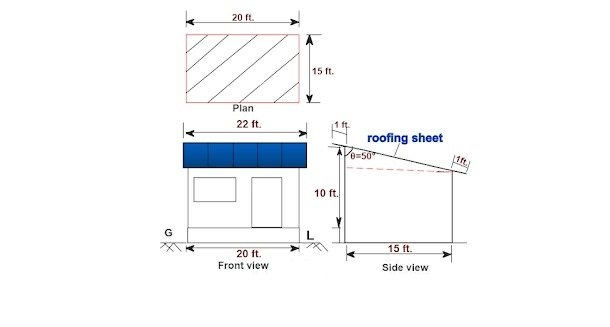### Given data:

Length of the building = 20 ft.

Width of the building = 15 ft.

Overhang on all four sides = 1ft

Angle θ = 50°

### Calculation:

First, we have to calculate the roofing length & width over the building.

### Roofing length

= [building length + overhang on 2 sides]

= [ 20 ft. + (2nos.× 1 ft.)]

### = 22 ft.

As you can observe in the above drawing, the sheet is sloped on the width side of the building.

Let us redraw the roofing triangle for a clear understanding.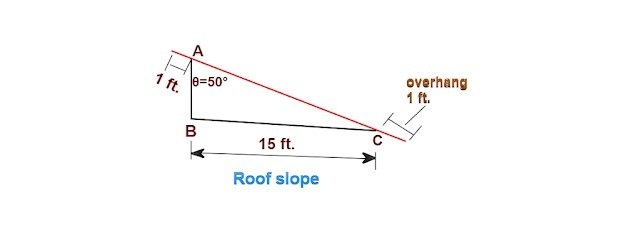Sloping length of the roofing

= [AC + (2nos. × overhang)]

### Now, let us calculate the length of AC as follows.

By trigonometry,

sinθ = opposite / hypotenuse

In the above triangle,

Side AC = hypotenuse

Side BC = opposite.

So,

Sin50° = [ 15 ft. ÷ hypotenuse]

0.766 = [ 15 ft. ÷ hypotenuse]

By Cross Multiplying,

Hypotenuse = [15 ft. ÷ 0.766 ]

2. ### Calculation For Road Work

The length of the roofing sheet

= [AC + (2nos. × overhang)]

=  [19.58 ft. + (2nos. × 1 ft.)]

### Sheet Calculation:

We will consider buying Tata dershane roofing sheets for this building.

Now, the effective width of this sheet after overlapping is shown below.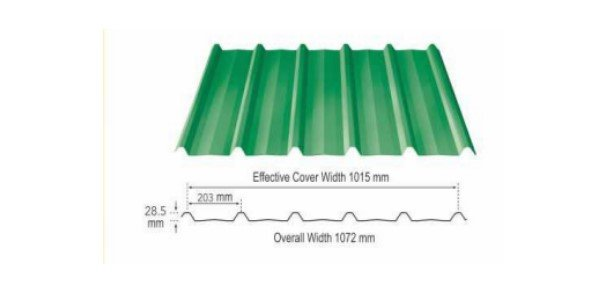### Given data:

Effective cover width We = 1015mm.

Total width Wt =  1072mm.

Roofing length Wr = 22 ft. ( calculated)

### The number of roofing sheets required for a single row

= [length side of the building for roofing ÷ effective width]

= [Wr ÷ We]

By converting the effective width unit into ft.

(1mm = 0.00328084ft.)

We = [1015mm × 0.00328084]

### Number of roofing sheets for a single row

= [22 ft ÷ 3.33 ft.]

### How To Calculate The Cost Of Modular Brick Wall Masonry With Full Detail

By rounding off the sheets, we need 7 numbers roofing sheets for a single row.

### b. Sheet calculation on width side of the building

Total required sloping length as calculated =21.58 ft.

Let us provide an overlapping of 250mm. ( 10″) between the two sheets.

So, the total length of sheets required

= [slopping length + overlapping ]

= [21.58 ft. + 0.833 ft.]

( as 10″ = 0.833 ft.)

### = 22.413 ft.

The available size of roofing sheets is shown below.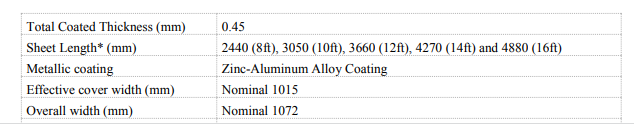### We will go for the 12 ft. length sheets for this building.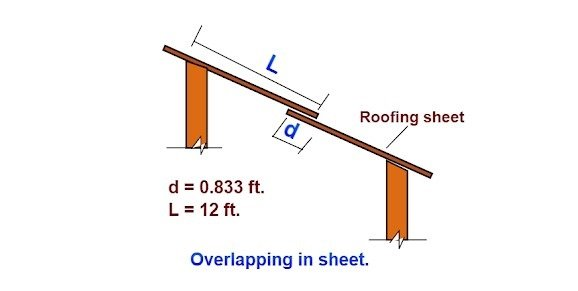Total sloping length after overlapping

= [ (2nos. × sheet length) – overlapping]

= [ ( 2nos. × 12 ft.) – 0.833 ft.]

23.167 ft. > 22.413 ft ✔ OK

So, we have to buy 14 nos. of 12 ft. length sheet for the roofing work of the building.

### Note:

1. We can buy 7 nos. of 12 ft. length sheet for one row & 7 nos. of 10 ft. length sheet for the second row. But, here we won’t get sufficient overlapping between the two rows.

## Land Surveying & Architects

### THANKS.